## 2.8  Vector Angular Momentum

The vector angular momentum of a particle is the cross product of the position and the linear momentum. For a rigid body the vector angular momentum is the sum of the vector angular momentum of each of the constituents. Here we find an expression for the vector angular momentum of a rigid body in terms of the inertia tensor and the angular velocity vector.

The vector angular momentum of a rigid body is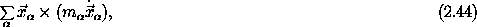where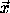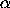,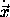, and mare the positions, velocities, and masses of the constituent particles. It turns out that the vector angular momentum decomposes into the sum of the angular momentum of the center of mass and the rotational angular momentum about the center of mass, just as the kinetic energy separates into the kinetic energy of the center of mass and the kinetic energy of rotation. As in the kinetic energy demonstration, decompose the position into the vector to the center of mass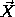and the vectors from the center of mass to the constituent mass elements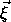: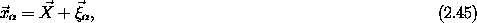with velocities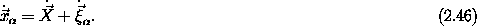Substituting, the angular momentum is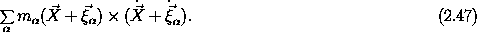Multiplying out the product, and using the fact thatis the center of mass and M = summis the total mass of the body, the angular momentum is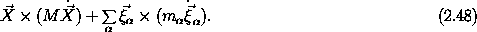The angular momentum of the center of mass is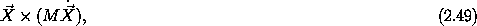and the rotational angular momentum is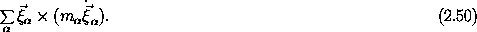We can also reexpress the rotational angular momentum in terms of the angular velocity vector and the inertia tensor, as we did for the kinetic energy. Using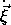=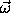×, we get the rotational angular momentum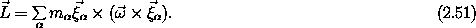In terms of components with respect to the basis {0,1,2}, this is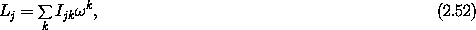where Ijk are the components of the inertia tensor (2.14). The angular momentum and the kinetic energy are expressed in terms of the same inertia tensor.

With respect to the principal-axis basis, the angular momentum components have a particularly simple form: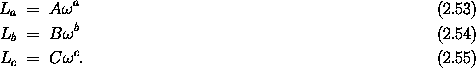Exercise 2.9.
Verify that expression (2.52) for the components of the rotational angular momentum (2.51) in terms of the inertia tensor is correct.

We can define procedures to calculate the components of the angular momentum on the principal axes:

(define ((Euler-state->L-body A B C) local)
(let ((omega-body (Euler-state->omega-body local)))
(column-matrix (* A (ref omega-body 0))
(* B (ref omega-body 1))
(* C (ref omega-body 2)))))

We then transform the components of the angular momentum on the principal axes to the components on the fixed basisi:

(define ((Euler-state->L-space A B C) local)
(let ((angles (coordinate local)))
(* (Euler->M angles)
((Euler-state->L-body A B C) local))))

These procedures are local state functions, like Lagrangians.